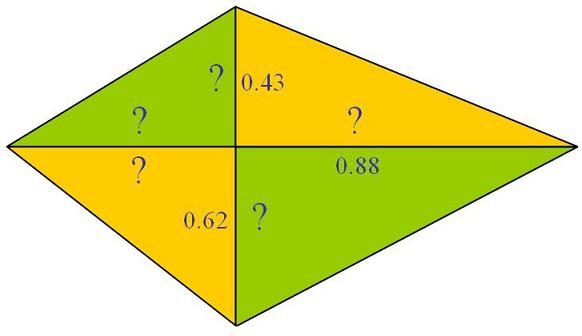#### You may also like### Golden Thoughts

Rectangle PQRS has X and Y on the edges. Triangles PQY, YRX and XSP have equal areas. Prove X and Y divide the sides of PQRS in the golden ratio.### At a Glance

The area of a regular pentagon looks about twice as a big as the pentangle star drawn within it. Is it?### Contact

A circular plate rolls in contact with the sides of a rectangular tray. How much of its circumference comes into contact with the sides of the tray when it rolls around one circuit?

# Eight Ratios

##### Age 14 to 16Challenge LevelTwo perpendicular lines lie across each other and the end points are joined to form a quadrilateral.

Eight ratios are defined, three are given but five need to be found.

Each original line is now in two parts and there are a further four lengths forming the quadrilateral.

The ratios work like this, 0.43 is the ratio between the upper portion of the vertical line and the hypotenuse of that orange triangle. Similarly 0.88 is the ratio between the right-hand portion of the horizontal line and the hypotenuse of that green triangle.

Can you find the five ratios which were not given?

This problem is about ratio, you could have a big version or a small version of the diagram and it would make no difference, so why not make the size something convenient ? Why not try having the hypotenuse of the top right orange triangle as your unit - ie. make that length 1 . What will you do next ?